# MAP 7th Grade Math : Geometry

## Example Questions

### Example Question #1 : Geometry

What is the area of the circle provided?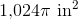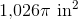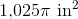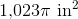Explanation:

In order to solve this problem, we need to recall the formula for the area of a circle: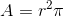The circle in this question provides us with the radius, so we can use the formula to solve: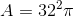Solve: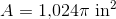### Example Question #1 : Geometry

The figure represents a set of complementary angles, solve for.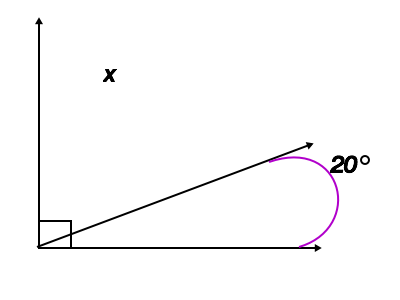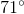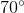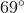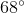Explanation:

Complementary angles are defined as two angles that when added together equalFrom the question, we know that the two angles are complimentary, and thus equal, so we can set up the following equation: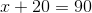Next we can solve for: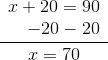### Example Question #3 : Geometry

Calculate the volume of the provided figure.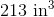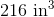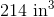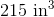Explanation:

In order to solve this problem, we need to recall the volume formula for a cube: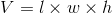Now that we have the correct formula, we can substitute in our known values and solve: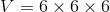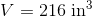### All MAP 7th Grade Math Resources